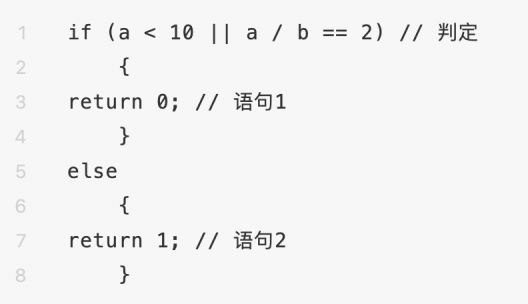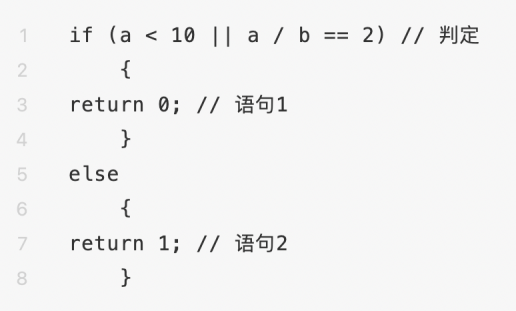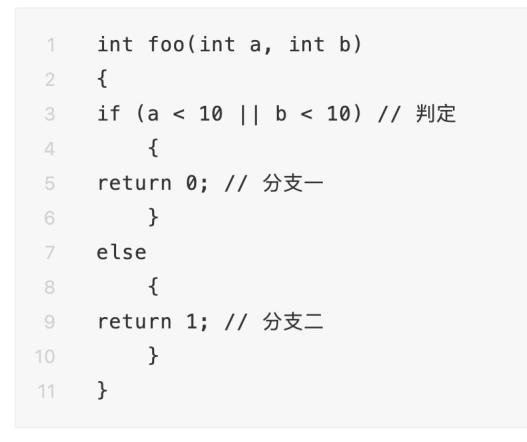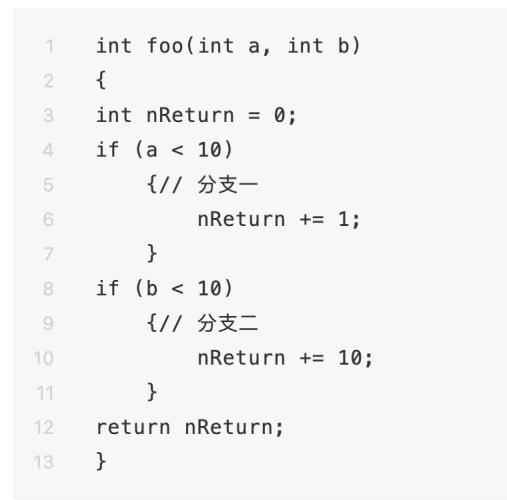TestCaes1: a=5，b＝任意数字

TestCaes2: a = 15，b =  15

完全的条件覆盖：

TestCase1: a = 5，b = 任意数字    ture X

TestCase2: a = 15， b = 5            false, true

TestCase2: a = 15， b = 15           false, falseTestCase a = 5，b = 5 ，nReturn = 11语句覆盖率100％

TestCase1 a = 5，b = 5，nReturn = 11

TestCase2 a = 15, b = 15， nReturn = 0

TestCase1 a = 5，b = 5， nReturn = 0

TestCase2 a = 15，b = 5 ，nReturn = 1

TestCase3 a = 5，b = 15，nReturn = 10

TestCase4 a = 15,   b = 15 ，nReturn = 11